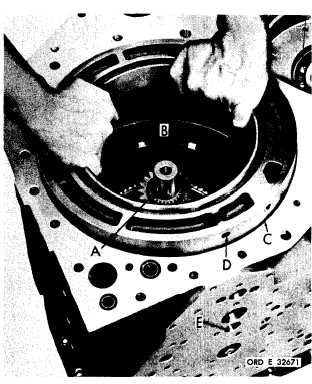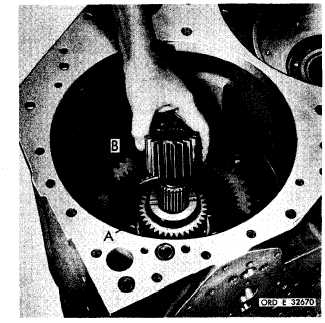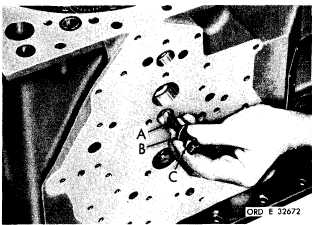Custom SearchC H A P    5,   SEC  X X X I II A S S E M B L Y PAR  227,   STEPS   1 - 14 Figure   250   (Step   11) I n s t a l l    i n t e r m e d i a t e - r a n g e    c l u t c h    b a c k    p l a te (A),   contour   side   up.   Install   Belleville   spring (B),   convex   side   up,   centering   it   on   plate   (A). Figure   251   (Step   12) Install    intermediate-range    sun    gear    (A),    flat side   down.   Install   low-range   sun   gear   (B). 1 6 6 Figure   252   (Step   13) Install   snap   ring   (A)   onto   turbine   shaft   (B). I n s t a l l    i n t e r m e d i a t e - r a n g e    p i s t o n    a n d    p i s t on housing   assembly   (C),   indexing   hole   (D)   with hole   (E). Figure   253   (Step   14) I n s t a l l    3 / 8 - 1 6    x    1 - 3 / 4    i n t e r m e d i a t e - r a n g e piston   housing   bolt   (A),   flat   washer   (B)   and l o c k    w a s h e r    ( C ) .    T i g h t e n    b o l t    o n l y    f i n g e r- tight   at   this   time.